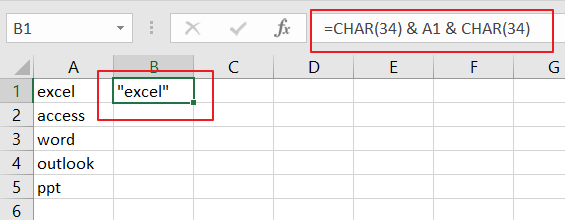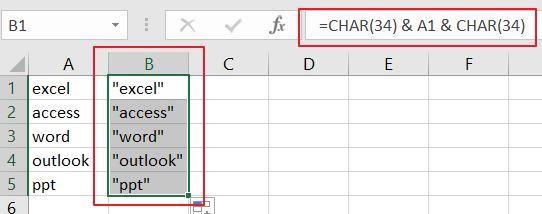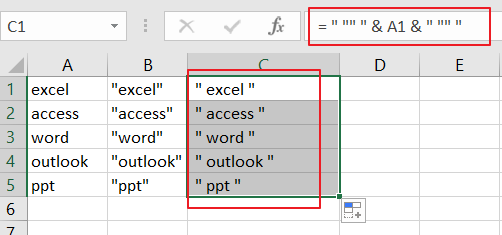# How to Add Quotes around Cell Values in Excel

This post will guide you how to add double quotes around cell values with a formula in your worksheet in Excel. How do I add quotes around each value of cells in Excel 2013/2016. How to add double quotes inside a formula in Excel.

Assuming that you have a long list of names that need to have quotes( it can be double or single quotes) around each name values. If there is a useful formula to add quotes to the name in each row or column.

## Adding Quotes around Cell Values

If you want to add quotes around text values for the specified cells or enclose all specified cell values with double quotes in range A1:A5, just do the following steps:

Step 1: you can type the following formula based on the CHAR function in Cell B1.

`=CHAR(34) & A1 & CHAR(34)`

or

`= " ' " & A1 & " ' "`Step 2: you need to drag the AutoFill handle in Cell B1 down to other cells to apply this formula. Then you would see that all the cell values have been enclosed with double quotes or single quotes.## Adding Quotes inside a Fromula

If you want to include double qutotes inside a formula in Excel, and you can use additional double qutoes as escape characters. For example, you wish to add double qutoes around a text, and you can also use the below formula:

`= " "" " & A1 & " "" "`### Related Functions

• Excel CHAR function
The Excel CHAR function returns the character specified by a number (ASCII Value). The syntax of the CHAR function is as below: =CHAR(number)….
Related Posts

How To Use Excel RRI Function

This post will guide you how to use Excel RRI function with syntax and examples in Microsoft excel. Description The Excel RRI function Returns an equivalent interest rate for the growth of an investment. So you can use the RRI ...

CAGR Formula Examples in Excel

CAGR in Excel is a formula that calculates the compound annual growth rate for any invested amount over the specified years or timeframe. Although there is no direct function in Excel that can help us identify the CAGR value, there ...

Repeating Character n Times in Excel

In daily life, we can use repeated characters to indicate the magnitude or priority of something. For example, a single “!” indicates a minor case, double “!!” indicates a medium case, and triple “!!!” indicates a high case, and so ...

Insert Line Break

If you have ever used MS Word or MS Powerpoint, you must be familiar that in them both, you can insert a line break by simply pressing the "Enter" key from your keyboard. We don't give any attention to this ...

Extract Multiple Lines From A Cell

Suppose that you have listed some text in a single cell which is separated by the line break(you can do it by pressing ALT + ENTER after entering the text), and now you want to extract multiple lines of text ...

Sidebar